# Importing Models from TensorFlow, PyTorch, and ONNX

The following post is from Sivylla Paraskevopoulou, Senior Technical Writer and David Willingham, Product Manager for Deep Learning Toolbox. How do you import a model created in TensorFlow™ or PyTorch™ and convert it into MATLAB Code?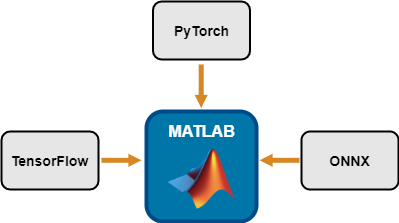First, keep in mind there are different options for working with deep learning models in MATLAB.
1. Using models created in MATLAB with Deep Learning Toolbox
2. Converting from other frameworks into MATLAB
3. Co-executing models from other frameworks with MATLAB
This blog post focuses on option 2, but you can see the benefits of choosing between these 3 options using this comparison table below, and view all available models to directly import into MATLAB using the MATLAB Deep Learning Model Hub. How complicated is it to convert a model? In many cases it's quite straightforward. In other cases, there may be a few additional steps before successfully converting a 3rd party model into MATLAB. The purpose of this blog is to give guidance on how to navigate these steps. Here is what we will cover today: Follow along step by step in this post, and download the code here: https://github.com/matlab-deep-learning/Image-Classification-in-MATLAB-Using-Converted-TensorFlow-Model

## Importing TensorFlow Models

It’s important to note that there are 2 different versions of TensorFlow models that can be imported:
• TensorFlow 2.0, where the SavedModel format is used
• TensorFlow 1.0, where the HDF5 format is used
(TensorFlow 2.0 supports both HDF5 and SavedModel formats, but the SavedModel format is recommended.) Your workflow in MATLAB will depend on the TensorFlow version in which the model was saved.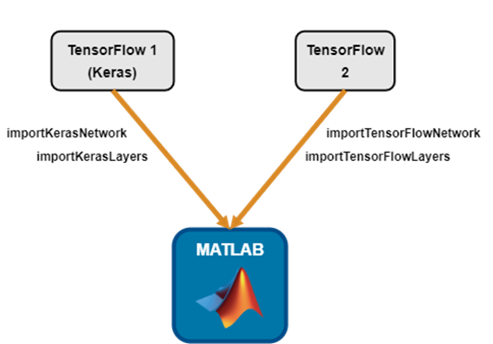Importing TensorFlow Models using SavedModel Format When TensorFlow 2.0 became available (Sep 2019), the SavedModel format was introduced and is now the preferred method for saving pretrained models. Here's the code to import this model format. Use tf.keras.applications.resnet_v2.ResNet50V2 to instantiate the ResNet50V2 model. The ResNet50V2 model is trained with images from the ImageNet database. Get the class names from SqueezeNet, which is also trained with ImageNet images.
squeezeNet = squeezenet;
ClassNames = squeezeNet.Layers(end).Classes;
Import ResNet50V2 in the SavedModel format by using the importTensorFlowNetwork function.
net = importTensorFlowNetwork("ResNet50V2",...
OutputLayerType="classification",...
Classes=ClassNames)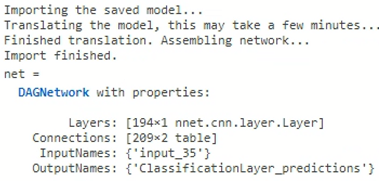Importing TensorFlow Models using the HDF5 (Keras) Format Import ResNet50V2 in the HDF5 format by using the importKerasNetwork function.
net = importKerasNetwork("ResNet50V2.h5",...
OutputLayerType="classification",...
Classes=ClassNames)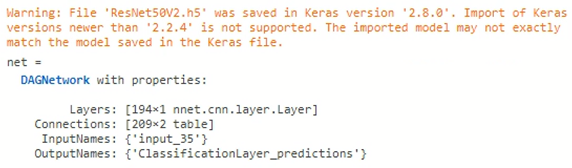The software throws a warning about the Keras version of the imported model. In the documentation page of each import and export function, there is a Limitations section where the supported TensorFlow or ONNX™ versions are described. For example, see Limitations of importTensorFlowNetwork.
 Tip: Even though you can successfully import ResNet50V2 with both importKerasNetwork and importTensorFlowNetwork, I recommend using importTensorFlowNetwork. Reasons for this become apparent when trying to import a more complex model with layers not supported for conversion into built-in MATLAB layers, as shown in the next section.

## Importing More Complex TensorFlow Models

This section shows how to import the TensorFlow model PNASNetLargeV4 which, unlike ResNet50V2, contains a complex feature-vector layer. This layer cannot be converted directly into a built-in MATLAB layer, so you'll need a custom layer. The good news is that MATLAB will autogenerate custom layers for you. To get the PNASNetLargeV4 model, run this code in Python®. You can find the code at https://tfhub.dev/google/imagenet/pnasnet_large/feature_vector/4.
import tensorflow as tf
import tensorflow_hub as hub
model = tf.keras.Sequential([
trainable=True),
tf.keras.layers.Dense(1000, activation='softmax')
])
model.build([None, 331, 331, 3])

The PNASNetLargeV4 model is trained with images from the ImageNet database. Importing using the HDF5 (Keras) Format (not recommended) When you import PNASNetLargeV4, the software cannot convert some of the TensorFlow layers into equivalent built-in MATLAB layers. First, try to import the model in HDF5 format by using the importKerasNetwork function.
net = importKerasNetwork("PNASNetLargeV4.h5",...
OutputLayerType="classification",...
Classes=ClassNames)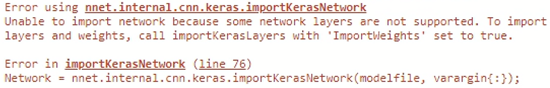The error message suggests using importKerasLayers to import the network architecture. importKerasLayers will import the model as a layer graph with placeholder layers. You must manually replace the placeholder layers to use the network for prediction or to retrain the network. You can replace a placeholder layer with a handwritten layer (documentation example: Assemble Network from Pretrained Keras Layers) or functionLayer (documentation example: Replace Unsupported Keras Layer with Function Layer).

### Importing using the SavedModel Format (recommended)

Alternatively, you can use the importTensorFlowNetwork function. It differs from its Keras equivalent because it has a unique feature; it can autogenerate custom layers. During importing, importTensorFlowNetwork generates custom layers in place of the TensorFlow layers that are not supported for conversion into built-in MATLAB layers.
net = importTensorFlowNetwork("PNASNetLargeV4",...
OutputLayerType="classification",...
Classes=ClassNames)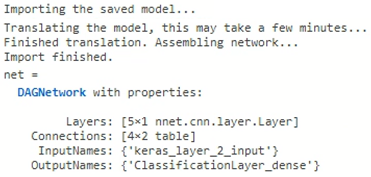Tip: A quick way to check if custom layers were generated for you is to inspect the package that was created during the import process.  E.g., for PNASNetLargeV4, the import function generates one large custom layer (kKerasLayer2Layer29666.m):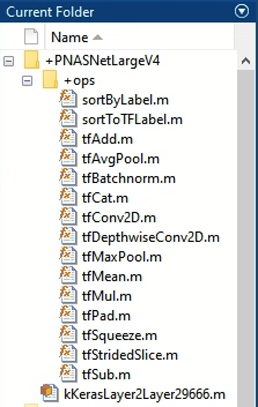### What if there is no SavedModel version of a model, just HDF5?

In this scenario, I would recommend to try and convert the TensorFlow model from HDF5 to saved model format. In most cases, this is the way to perform the conversion from within TensorFlow: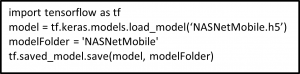However, you might encounter a use case that requires "model surgery". For example, if an unsupported layer is written in the older version, and you attempt to save it in the newer SavedModel version, TensorFlow may throw an error stating that it doesn't know how to convert the model. In this situation you must do one of the following:
1. Manually re-code the layer in the newer TensorFlow SavedModel format prior to importing the model into MATLAB.
2. Import the older model into MATLAB using importKerasLayers. It will create a placeholder layer in place of the custom layer, which the software could not import. Then, from within MATLAB you manually re-code the layer.
Both options require you to write layers in either TensorFlow (option 1) or MATLAB (option 2).

## Importing PyTorch Models

Currently, Deep Learning Toolbox does not support importing models directly from PyTorch; However, you can import the model via ONNX.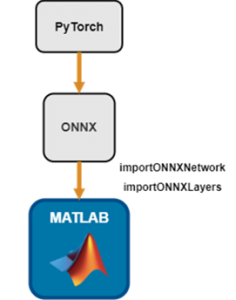First, you need to convert the PyTorch model to the ONNX model format by following the instructions in Exporting a Model from PyTorch to ONNX. Then, you can import the ONNX model into MATLAB by using importONNXNetwork. Here, we show you how to import the squeezenet.onnx model, which you can also find in the PyTorch Model Zoo.
net = importONNXNetwork("squeezeNet.onnx");
The importONNXNetwork function can also generate custom layers when the software cannot convert ONNX operators into equivalent built-in MATLAB layers. Import the shufflenet-9.onnx model with autogenerated custom layers. By default, importONNXNetwork returns a DAGNetwork object that is ready to use for prediction.
net = importONNXNetwork("shufflenet-9.onnx",PackageName="shuffleNet");
importONNXNetwork saves the custom layers in the package +shuffleNet, in the current folder, similarly to importTensorFlowNetwork. You can also export a trained Deep Learning Toolbox network to the ONNX model format by using the exportONNXNetwork function.
exportONNXNetwork(net,"myNet.onnx")

# Example: Import Complex TensorFlow Model for Image Classification

This example shows how to import a pretrained TensorFlow model in the SavedModel format, and use the imported network to classify an image. The TensorFlow model contains layers that are not supported for conversion into built-in MATLAB layers. The software automatically generates custom layers when you import these layers.

## Import Pretrained TensorFlow Model

Use tf.keras.applications.efficientnet_v2.EfficientNetV2L to instantiate the EfficientV2L model. The EfficientNetV2L model is trained with images from the ImageNet database. Get the class names from SqueezeNet, which is also trained with ImageNet images.
squeezeNet = squeezenet;
ClassNames = squeezeNet.Layers(end).Classes;
Import the TensorFlow model EfficientNetV2L in the SavedModel format by using the importTensorFlowNetwork function. Specify the output layer type for an image classification problem.
net = importTensorFlowNetwork("EfficientNetV2L",...
OutputLayerType="classification",...
Classes=ClassNames);
Find the autogenerated custom layers.
PackageName = '+EfficientNetV2L';
s = what(['.\' PackageName]); %'
ind = zeros(1,length(s.m));

for i = 1:length(net.Layers)
for j = 1:length(s.m)
if strcmpi(class(net.Layers(i)),[PackageName(2:end) '.' s.m{j(1:end-2)])
ind(j) = i;
end
end
end

display(ind)
ind = 2
display(net.Layers(ind))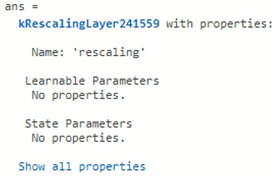Analyze the imported network.
analyzeNetwork(net)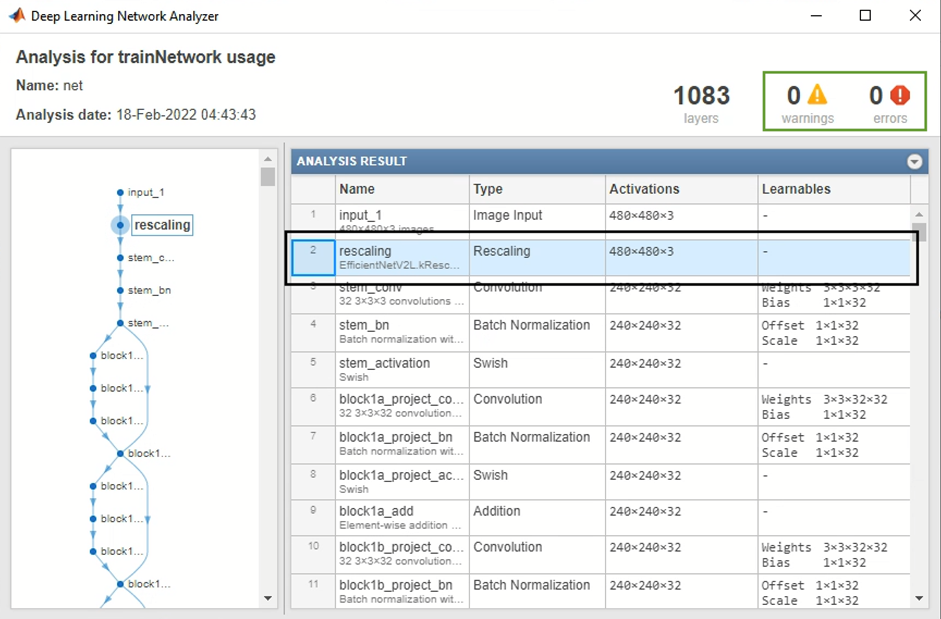As expected, the imported network, which contains autogenerated custom layers, shows no warnings or errors in the network analyzer. This means the imported network is ready to use for prediction.

## Read and Preprocess Input Image

TensorFlow provides the tf.keras.applications.efficientnet_v2.preprocess_input method to preprocess image input data for the EfficientNetV2L model. Here, we replicate the input preprocessing by resizing, rescaling, and normalizing the input image. Read the image you want to classify and display the size of the image. The image is 792-by-1056 pixels and has three color channels (RGB).
Im = imread("peacock.jpg");
size(Im)
Resize the image to the input size of the network.
InputSize = net.Layers(1).InputSize;
Im = imresize(Im,InputSize(1:2));
The inputs to  EfficientNetV2L require further preprocessing. Rescale the image. Normalize the image by subtracting the training images mean and dividing by the training images standard deviation. To find the values that you should use, see https://github.com/keras-team/keras-applications/blob/master/keras_applications/imagenet_utils.py.
ImProcessed = rescale(Im,0,1);
meanIm = [0.485 0.456 0.406];
stdIm = [0.229 0.224 0.225];
ImProcessed = (ImProcessed-reshape(meanIm,[1 1 3]))./reshape(stdIm,[1 1 3]);

## Classify Image Using the Imported Network

Classify the image using the imported network. Show the image with the classification label.
label = classify(net,Im);
imshow(Im)
title(strcat("Predicted label: ",string(label)))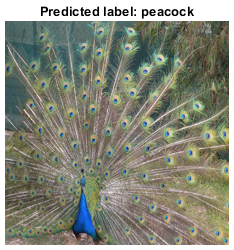You can also use the imported network with the Predict block of the Deep Learning Toolbox, to classify an image in Simulink. For an example, see the previous post: Bringing TensorFlow Models into MATLAB. The fact that the imported network contains autogenerated custom layers doesn’t hinder modeling in Simulink.

# Summary

Interoperability with deep learning frameworks, such as TensorFlow and PyTorch, enables you to do more in MATLAB and Simulink. It gives the flexibility to take full advantage of the MATLAB ecosystem and integrate it with resources developed by the open-source community. It also enables you to combine workflows that include data-centric preprocessing, model tuning, model compression, model integration, and automatic code generation for models developed outside MATLAB. Comparison of capabilities for working with deep learning models in MATLAB
 Capability Models created using Deep Learning Toolbox Models Converted from other FrameWorks Co-Execution Integrates with pre and post processing in MATLAB Requires installation of products only Supports debugging from MATLAB Inference performance in MATLAB and Simulink Available MATLAB application examples Requires no datatype conversion and data reformatting Coverage for embedded code generation with MATLAB Coder, GPU Coder & Deep Learning HDL Toolbox Requires no additional libraries for standalone deployment from MATLAB Compiler Access popular models in a single line of code Access to open-source models from TensorFlow and PyTorch
 Most support Limited support No support

You must have support packages to run the import and export functions in Deep Learning Toolbox. If the support package is not installed, each function provides a download link to the corresponding support package in the Add-On Explorer. A recommended practice is to download the support package to the default location for the version of MATLAB you are running. You can also directly download the support packages from File Exchange. Table: Support packages required to run import and export functions. Table: Comparing high-level features of the three functions that can import deep learning networks.
 importTensorFlowNetwork importKerasNetwork importONNXNetwork Support for TensorFlow ✅* ✅** Support for PyTorch ✅ Support for autogeneration of custom layers ✅ ✅
* Currently supports TensorFlow versions 2.0-2.6 (thoroughly tested). In most cases, models saved in newer TensorFlow versions are also importable. Requires Deep Learning Toolbox Converter for TensorFlow Models ** Supports TensorFlow-Keras versions up to 2.2.4, with limited support for versions 2.2.5 to 2.4.0. Requires Deep Learning Toolbox Converter for TensorFlow Models The software in the support packages is updated monthly. These monthly updates can include new layer support for import and export, updated TensorFlow and ONNX version support, and bug fixes. Major features are released with the general release.

Published with MATLAB® R2020b

|